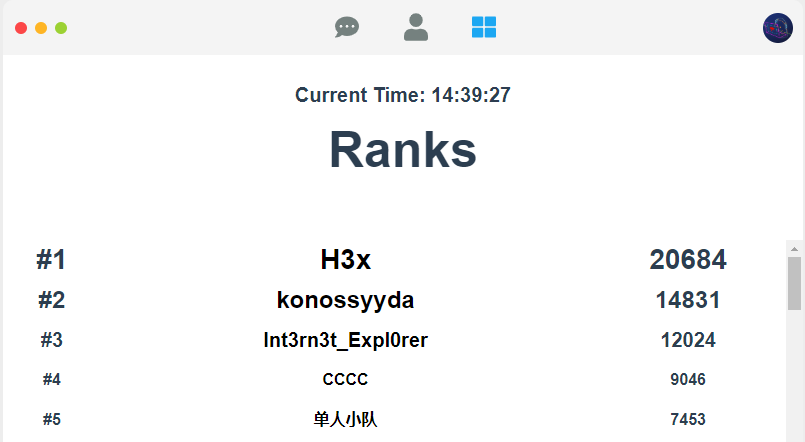### Starting Point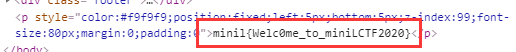## MISC

### MiniGameHacking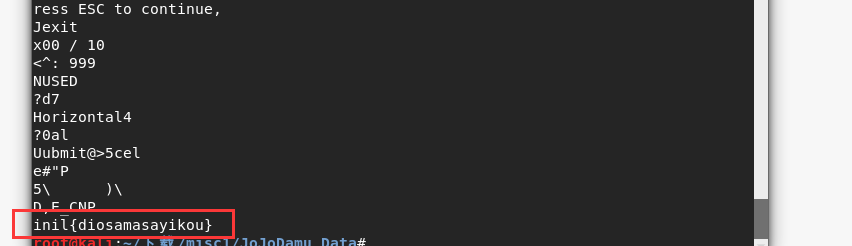### EasyVmem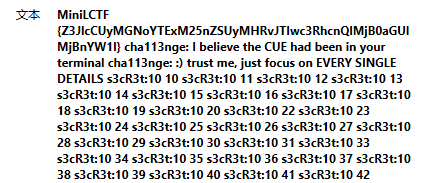``volatility -f challenge.vmem --profile=Win7SP1x64 clipboard -v > out.txt``

``````import binascii

out = ''
f = open('out.txt','r')
fi = open('res.txt','w')
while 1:
if a:
b = a.split('  ').replace('00', '').replace(' ', '')
b = binascii.unhexlify(b)
out += b
else:
break

fi.write(out)
fi.close()``````

``````from PIL import Image

img = Image.new('RGB', (400, 400), (0, 0, 0))
f = open('res.txt','r')
while 1:
if a:
x, y = a[7:].split(' ')
x = int(x)
y = int(y)
img.putpixel((x, y), (255, 255, 255))
else:
break

img.save('flag.png')``````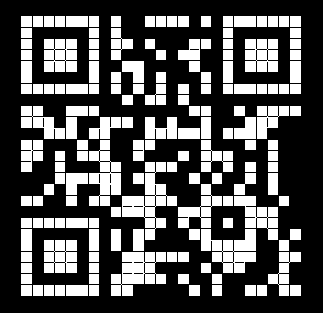## ANDROID

### TestOnly?

• 有两个面对不同对象的a函数，一个b函数，一个I函数（没啥用），一个J函数（得到flag）
• 第一个a函数面向字符（char类），第二个a函数面向字符串（String类）
• b函数面向字符串，将字符串SHA1后`hexdigest()`
• J函数创建了一个数组，与localObject进行按位异或操作得到flag

``````from hashlib import sha1

flag = ''
loc = b'B08020D0FACFDAF81DB46890E4040EDBB8613DA5ABF038F8B86BD44525D2E27B26E22ACD06388112D8467FD688C79CC7EA83F27440577350E8168C2560368616'
loc = sha1(loc).hexdigest().encode()
arr = [85,95,5,83,75,96,94,0,17,61,102,87,80,123,4,105,85,83,101,109,55,85,23,48,106,1,40,7,97,31]
for i in range(len(arr)):
flag += chr(arr[i] ^ loc[i])

print(flag.replace('flag','minil'))``````